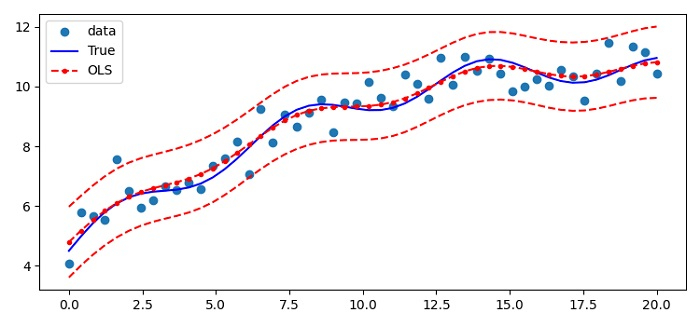# How to plot statsmodels linear regression (OLS) cleanly in Matplotlib?

MatplotlibPythonData Visualization

We can plot statsmodels linear regression (OLS) with a non-linear curve but with linear data.

## Steps

• Set the figure size and adjust the padding between and around the subplots.

• To create a new one, we can use seed() method.

• Initialize the number of sample and sigma variables.

• Create linear data points x, X, beta, t_true, y and res using numpy.

• Res is an ordinary Least Square class instance.

• Calculate the standard deviation. Confidence interval for prediction applies to WLS and OLS, not to general GLS, that is, independently but not identically distributed observations.

• Create a figure and a set of subplots using subplot() method.

• Plot all the curves using plot() method with (x, y), (x, y_true), (x, res.fittedvalues), (x, iv_u) and (x, iv_l) data points.

• Place the legend on the plot.

• To display the figure, use show() method.

## Example

import numpy as np
from matplotlib import pyplot as plt
from statsmodels import api as sm
from statsmodels.sandbox.regression.predstd import wls_prediction_std
plt.rcParams["figure.figsize"] = [7.50, 3.50]
plt.rcParams["figure.autolayout"] = True
np.random.seed(9876789)
nsample = 50
sig = 0.5
x = np.linspace(0, 20, nsample)
X = np.column_stack((x, np.sin(x), (x - 5) ** 2, np.ones(nsample)))
beta = [0.5, 0.5, -0.02, 5.]
y_true = np.dot(X, beta)
y = y_true + sig * np.random.normal(size=nsample)
res = sm.OLS(y, X).fit()
prstd, iv_l, iv_u = wls_prediction_std(res)
fig, ax = plt.subplots()
ax.plot(x, y, 'o', label="data")
ax.plot(x, y_true, 'b-', label="True")
ax.plot(x, res.fittedvalues, 'r--.', label="OLS")
ax.plot(x, iv_u, 'r--')
ax.plot(x, iv_l, 'r--')
ax.legend(loc='best')
plt.show()

## Output